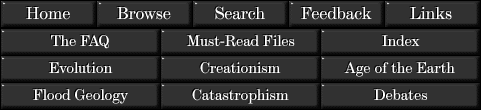# How Old is the Earth

## A Response to “Scientific” Creationism### Addendum: Derivation of the Neutron Reaction Correction Equation†

Jon Fleming has added this section in 2005. The purpose is to show the derivation of Cook’s “correction” equation and how non-equal cross-sections affect the result. It is based on two major premises, both of which are almost certainly false:

1. There is no primordial lead in the samples under consideration; all lead was created by radioactive decay and/or neutron capture.

2. The neutron flux was sufficient to create all the 208Pb in the samples, since the 208Pb could not have come from the decay of 232Th (which would leave at least a little residual 232Th in the samples today).

Recall the neutron-capture reaction series presented in the main text: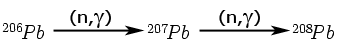We’ll move from simple to more complex; starting with the end of the chain, then considering the beginning of the chain, and finally looking at the middle of the chain.

Since we “know” that all the 208Pb was generated by neutron capture, using C207→208 to indicate the cross section for converting 207Pb to 208Pb by neutron capture, using N to denote the neutron flux and using 208Pbmeasured to denote the measured amount of 208Pb in the sample today: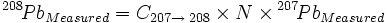(1)

Or, solving for N: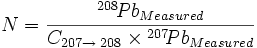(2)

Next we move to the beginning of the chain. Here there is one mechanism creating 206Pb (radioactive decay of 238U) and another mechanism destroying 206Pb (neutron capture). Therefore the measured amount of 206Pb today is less than the amount created from decay of 238U. Using 206PbRadiogenic as the 206Pb that was generated from radioactive decay and should be used in the determination of age; 206PbMeasured as the 206Pb that was actually measured in the sample “today”; and 206PbConverted as the 206Pb that was generated from radioactive decay and then was converted to 207Pb by neutron capture: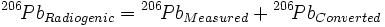(3)

206PbConverted is, of course, the amount of 206Pb measured times a conversion factor that is the product of the cross section (for converting 206Pb to 207Pb by neutron capture) and the total neutron flux. Since the 206Pb and 207Pb were in close proximity, the total neutron flux N is the same for both. Calling the cross section C206→207: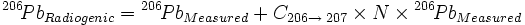(4)

Since N is the same for both 206Pb and 207Pb, we can substitute the right-hand side of equation (2) for the “N” term in equation (4):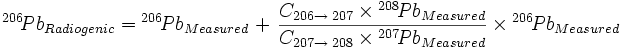(5)

Now we consider the middle of the neutron capture series, the amount of 207Pb generated from radioactive decay, 207PbRadiogenic, which should be used in the calculation of age. Here two mechanisms are creating 207Pb (radioactive decay of 235U and neutron capture of 206Pb) and one mechanism is destroying 207Pb (neutron capture creating 208Pb).

207PbRadiogenic is the measured amount 207PbMeasured plus the amount of 207Pb that was generated by radioactive decay but then was converted to 208Pb by neutron capture (which in turn is equal to the amount of 208PbMeasured since we “know” that all the 208Pb came from a one-to-one conversion of 207Pb) and then minus the amount of 207Pb that was generated from 206Pb by neutron capture rather than radioactive decay (which in turn is the second term of the right-hand side of equation (5)):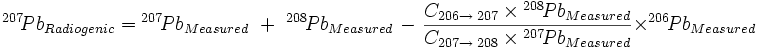(6)

And, finally, dividing equation (5) by equation (6):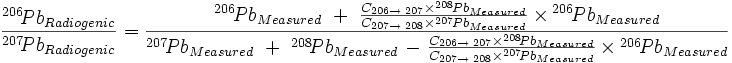(7)

which, when C207→208 = C206→207 (as Cook assumed), reduces to the equation given in the main text except for the way that the Pb terms are grouped in the last term of both the numerator and denominator: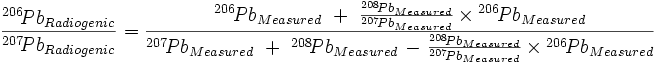(8)

For calculating the 206Pb/207Pb for the third row of Table 5, using Dalrymple’s values for cross sections plugged into equation (7):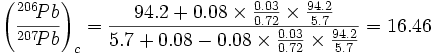I do not know why my result is slightly (0.5%) different from Dalrymple’s 16.38, but the difference is not significant.

Using more recent values for the cross sections: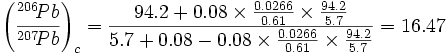which is insignificantly different from the other values.

To calculate the age from these “corrected” values of 206Pb/207Pb , we use the standard equation (which cannot be solved in closed form) for age “t” of a sample (in years) given its 206Pb/207Pb ratio, the decay constant of 238U (λ1 = 1.55125×10-10 per year), and the decay constant of 235U (λ2 = 9.8485×10-10 per year) is: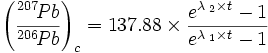(from Dalrymple, G. Brent, “The Age Of the Earth”, Stanford University Press, 1991, page 101. The values of λ1 and λ2 are from the same source, page 80. Note that the equation uses 207Pb/206Pb, the inverse of the lead ratios used in this paper and addendum)

The age equation is easily solved by any of a variety of numerical techniques. For 206Pb/207Pb = 16.46 the calculated age is 630 million years, and for 206Pb/207Pb = 16.47 the calculated age is 629 million years. Including the effect of non-equal cross-sections for the neutron capture reactions completely obviates Cook’s conclusion. Neutron capture does not noticeably affect the measurement of ages by 206Pb/207Pb ratios.